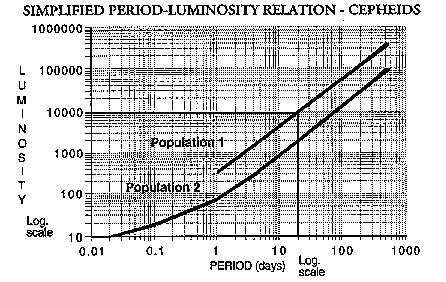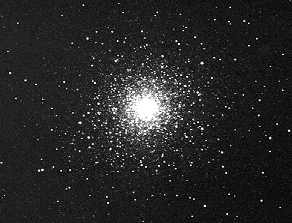# To find the distance of a Cepheid

Let us use the Cepheid method to find the distance of a star cluster. Suppose we have observed a Population 1 Cepheid in the cluster with a period of 11 days and average apparent magnitude of 2.3.From the graph we see that the star's luminosity must be 10 000 times that of the Sun. This corresponds to a magnitude difference of 10. Click here for an explanation of the magnitude system. Since the Sun's absolute magnitude is 4.8, the absolute magnitude of the star must be
4.8 - 10 = -5.2
We can now use a simple equation connecting absolute and apparent magnitude with distance to find that the distance of the star and also its cluster is about 320 parsecs.Cepheids in globular clusters like this (M92) are used to help map our galaxy.